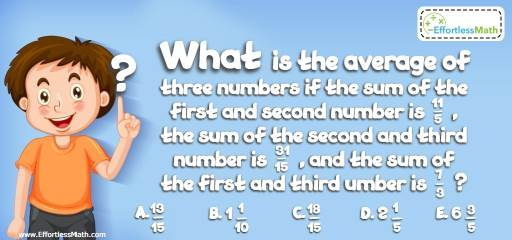# Algebra Puzzle – Challenge 40

This is a perfect mathematics puzzle for those who enjoy solving hard math and critical thinking challenges. Let's challenge your brain!## Challenge:

What is the average of three numbers if the sum of the first and second number is $$\frac{11}{5}$$, the sum of the second and third number is $$\frac{31}{15}$$, and the sum of the first and third umber is $$\frac{7}{3}$$?

A- $$\frac{13}{15}$$

B- $$1\frac{1}{10}$$

C- $$\frac{18}{15}$$

D- $$2\frac{1}{5}$$

E- $$6\frac{3}{5}$$

### The Absolute Best Book to challenge your Smart Student!

Let X, Y and Z represent the three numbers. Therefore:
$$X + Y = \frac{11}{5}, Y + Z = \frac{31}{15}, X + Z = \frac{31}{15}$$
$$(X + Y) + (Y + Z) + (X + Z) = \frac{11}{15} + \frac{31}{15} + \frac{7}{3}$$
$$2X + 2Y + 2Z = \frac{99}{15} → 2 (X + Y + Z) = \frac{99}{15} → X + Y + Z = \frac{99}{30 }= \frac{33}{1}$$
The average of the three numbers is:
$$\frac{X + Y + Z}{3 }= \frac{33}{10} ÷ 3 = \frac{33}{30} = \frac{11}{10}$$

### What people say about "Algebra Puzzle – Challenge 40 - Effortless Math: We Help Students Learn to LOVE Mathematics"?

No one replied yet.

X
30% OFF

Limited time only!

Save Over 30%

SAVE $5 It was$16.99 now it is \$11.99